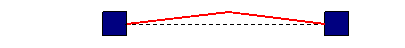# Waves Homework Problems

Michael Fowler  3/29/07

1. Sketch the appearance of a single transverse wave pulse traveling down a string at some instant, and below it sketch the velocities of the small segments of string at that same instant.

2.  Given that the note middle C corresponds to a frequency of 262 Hz., find the length of an organ pipe having this as its fundamental (lowest frequency) note

(a) if both ends of the pipe are open

(b) if one end is closed, one end open.

(c) What note would the organ pipe sound if filled with helium gas? (the bulk modulus is the same as for air).

3. Humans can only hear sounds in the frequency range 20 Hz to 20,000 Hz.  What would be the longest and shortest organ pipes there is any point in manufacturing?

4.  The speed of sound in water is about 1.5 km/sec.

(a)  Figure out from that the bulk modulus of water.

(b) How compressed in volume is the water at the bottom of the deepest ocean?

5. A piston (a speaker) at one end of a long tube is oscillating at 262 Hz with an amplitude of 0.2mm.  The piston is circular, with a diameter 5 cm.

(a) Find the maximum pressure variation at the plate.

(b) What is the average power output of the plate?

(c) How much energy is there in one meter of the traveling wave as it goes down the tube?

6. Assume a steel piano wire is 50 cm long and weighs 3 grams.  It is held at a tension of 1,000N.  (a)  What is its fundamental frequency?

(b)  What is the wavelength of that sound in air?

(c) How does the speed of a traveling wave in the steel piano wire compare to the speed of sound in air?  The speed of sound in solid steel is far greater than the speed of sound in air.  Does that contradict your finding for the wire?  Explain why or why not.

7. A string under tension is pulled aside in the middle so it has the following V-shape:Describe with a series of sketches the subsequent motion of the string.

(Hint: study the spreadsheet addition of two traveling sine waves to form a standing wave. The initial string configuration here is half a wave length of a zigzag wave.)